# Amines – Organic Chemistry – list of solved problems

Go to the page with the solutions to the problems.

## Amines – list of problems

### Problem 1)

Classify the following compounds (a-h) as primary amines, secondary amines, tertiary amines and quaternary ammonium salts, as appropriate.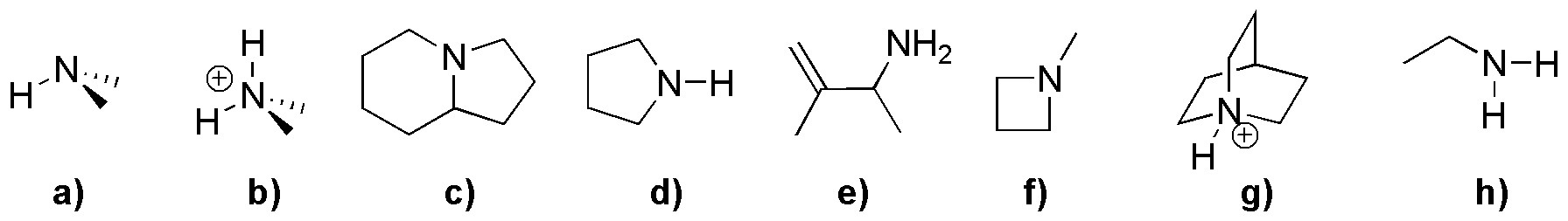### Problem 2)

Complete the following Gabriel syntheses (a-c).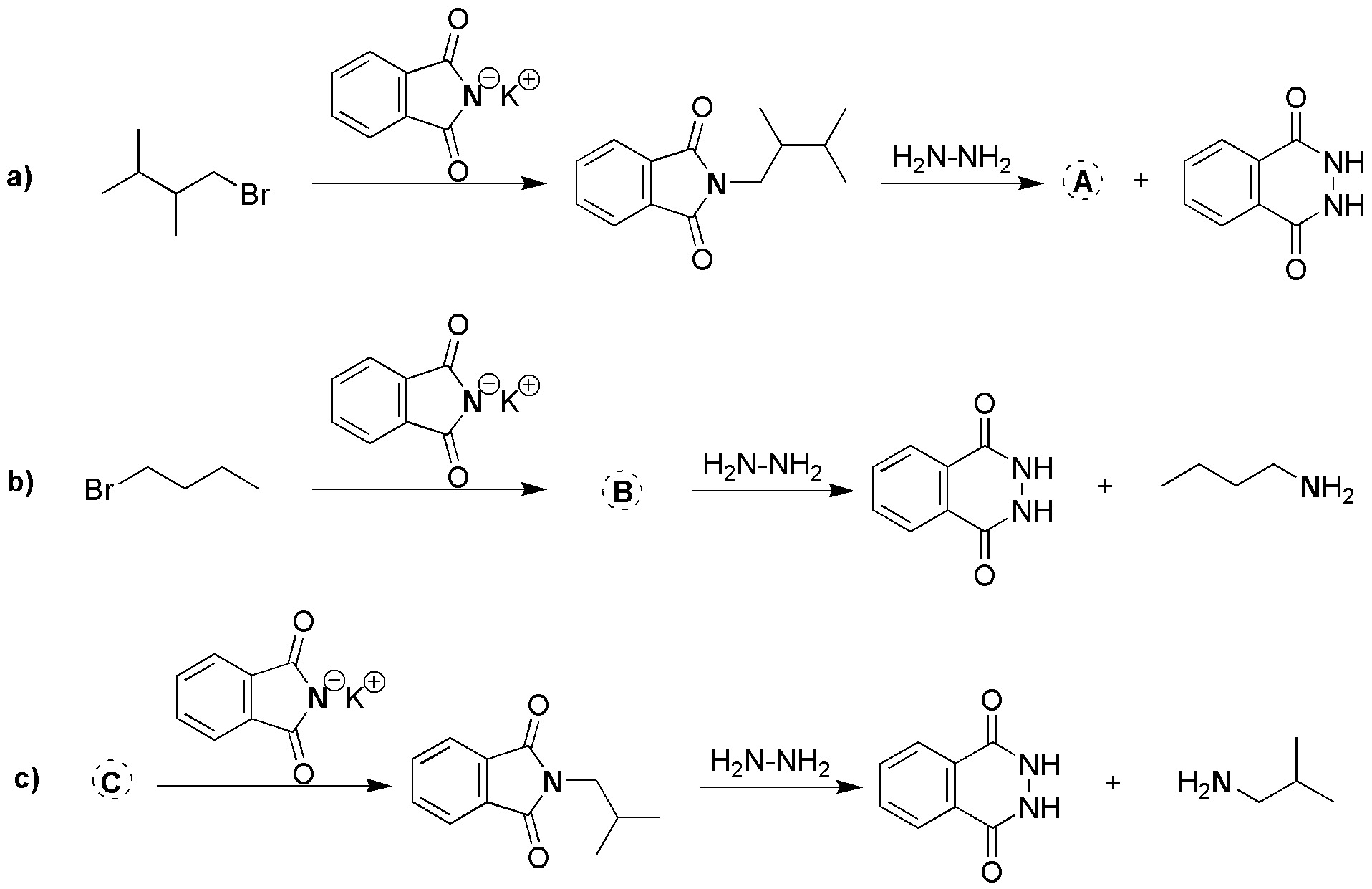### Problem 3)

Order in increasing order of basicity the following compounds, justifying the results.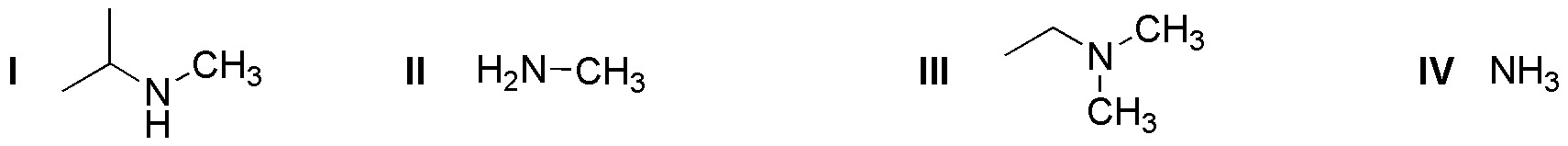### Problem 4)

a) Order from higher to lower basicity the following amines, justifying the result.

• (I) Methylamine
• (II) Aniline
• (III) p-Chloroaniline
• (IV) Trimethylamine
• (V) Tetramethylammonium bromide

b) Which amine in the series will be most effective as a nucleophile?

### Problem 5)

Describe a simple method to obtain N-methylbenzamide from methylamine.

### Problem 6)

When pentyl amine is treated with nitrous acid in the presence of hydrochloric acid, nitrogen is observed to be given off and a mixture of 5 products is formed. Justify the result.

### Problem 7)

An amine of molecular formula C6H15N reacts with one mole of CH3I followed by hot wet silver oxide to give but-1-ene. Deduce which amine it is.

### Problem 8)

An aqueous solution of sodium hydroxide and benzenesulfonyl chloride is reacted with an amine of molecular formula C4H11N to give a precipitate. From this result deduce the structure of the amine.

### Problem 9)

Complete the following reactions (a-d):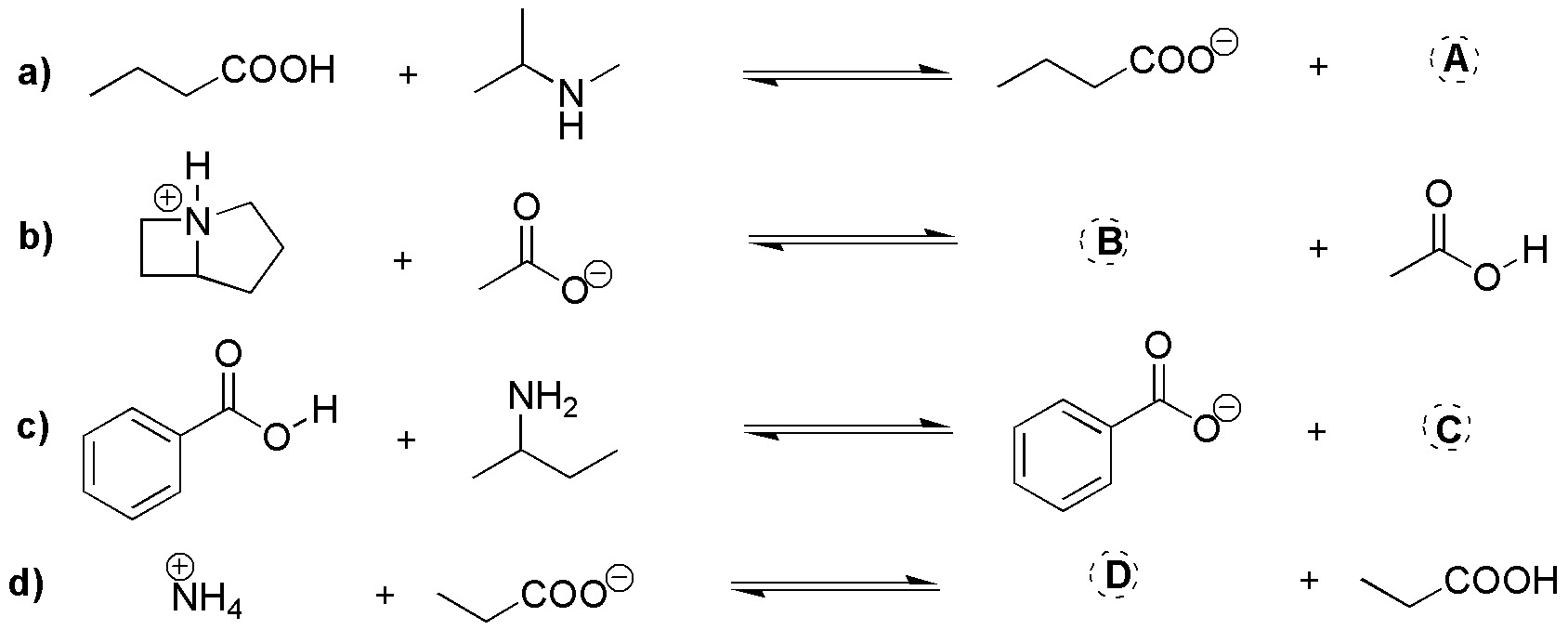### Problem 10)

Normally, the alkylation of a primary or secondary amine does not stop at monoalkylation because the product reacts back with the excess of the amine used as a reactant. Write all the products for the alkylation reaction between ethylamine and methyl iodide.

### Problem 11)

Justify whether amines (a-f) can be obtained by a Gabriel synthesis.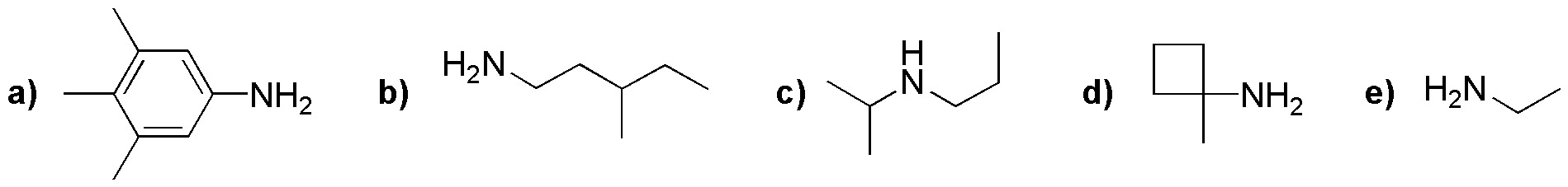### Problem 12)

What reagents (a-f) are needed to obtain the following compounds (I-III) by the Mannich reaction?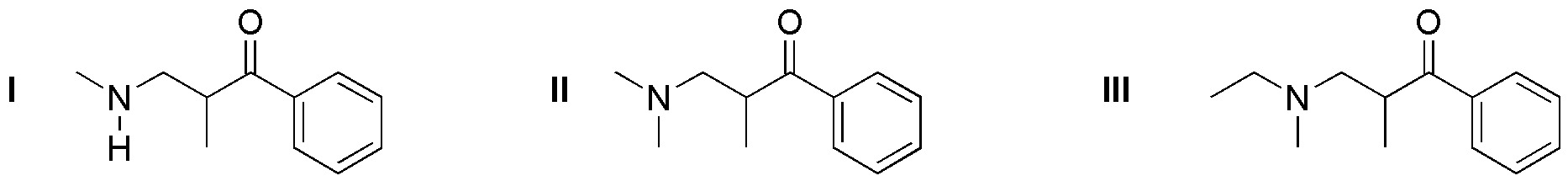• a) CH3CH2NHCH3 + CH2O + HCOPh
• b) (CH3)3N + CH3CH2COPh
• c) CH3CH2NHCH3 + CH2O + CH3CH2COPh
• d) CH3CH2CH2N(CH3CH2)CH3 + HCOPh
• e) CH3NH2 + (CH3)2CHCOPh
• f) CH3CH2N(CH3)2 + CH2O + HCOPh
• g) (CH3)2NH + (CH3)2CHCOPh
• h) CH3CH2N(CH3)2 + CH3CH2COPh
• i) (CH3)2NH + CH3CH2COPh
• j) CH3CH2N(CH3)2 + CH2O + CH3COPh
• k) CH3CH2CH2N(CH3)2 + HCOPh
• l) (CH3)2NH + CH2O+ CH3COPh
• m) CH3NH2 + CH2O + CH3CH2COPh
• n) (CH3)3N + CH2O + CH3COPh
• o) (CH3CH2)2NCH3 + CH2O + HCOPh
• p) (CH3)2NH + CH2O+ CH3CH2COPh
• q) CH3CH2NHCH3 + (CH3)2CHCOPh
• r) CH3CH2CH2NHCH3 + HCOPh

### Problem 13)

Write which intermediate and major product is obtained in the following sequences of reactions ending with Hofmann elimination.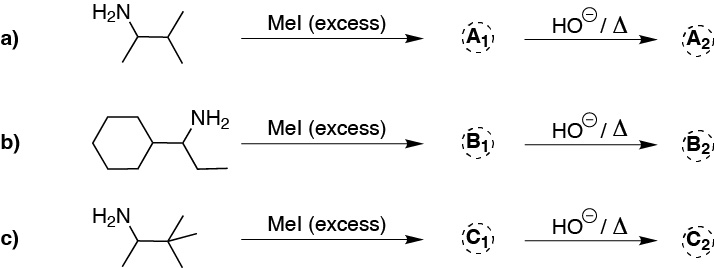### Problem 14)

Classify the following compounds (a-h) as primary amines, secondary amines, tertiary amines and quaternary aminium salts.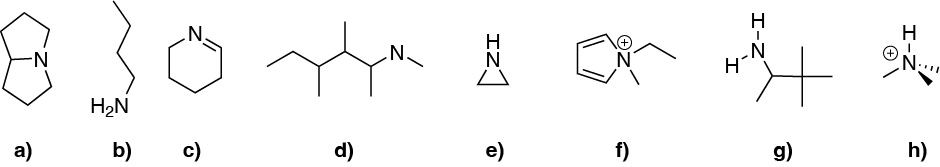### Problem 15)

Complete the following acid-base reactions of amines (a-d).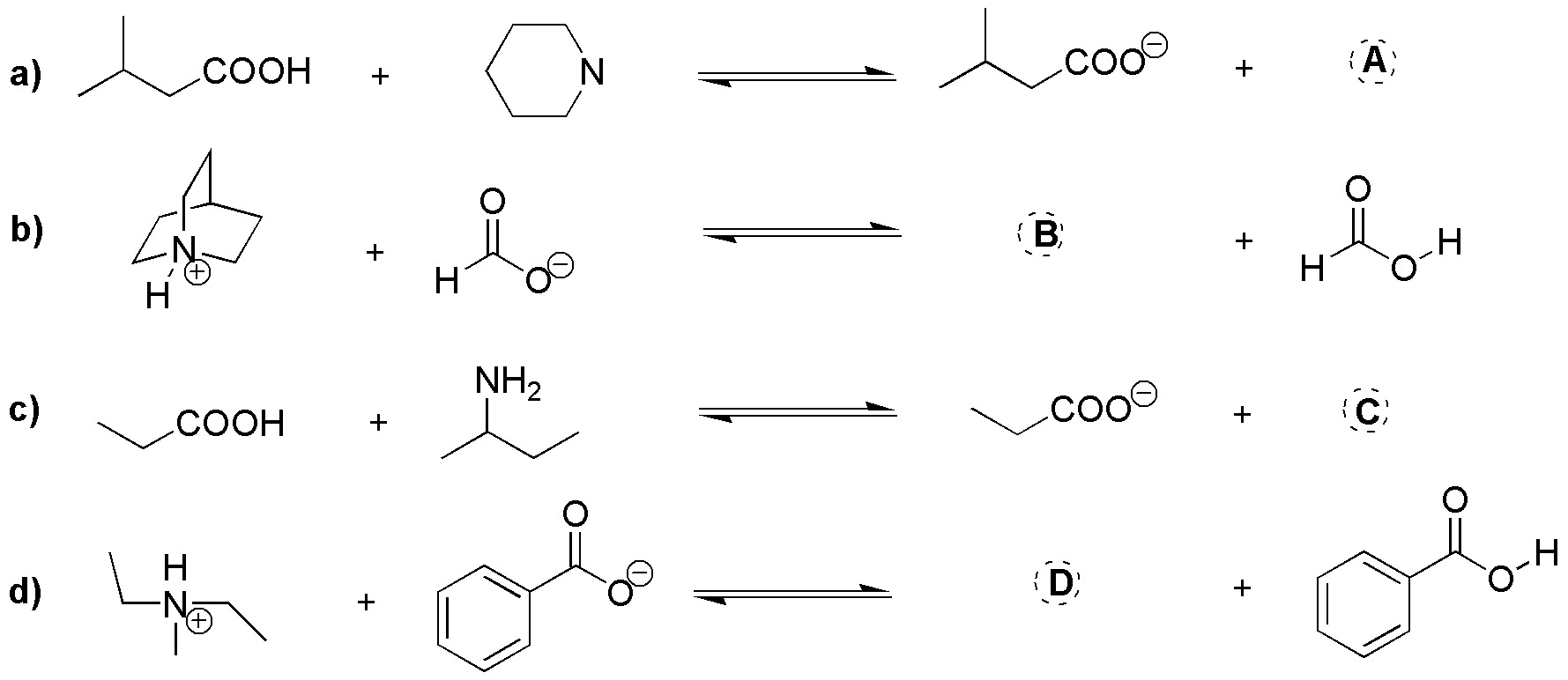### Problem 16)

Write the major product obtained in the second Hofmann elimination steps.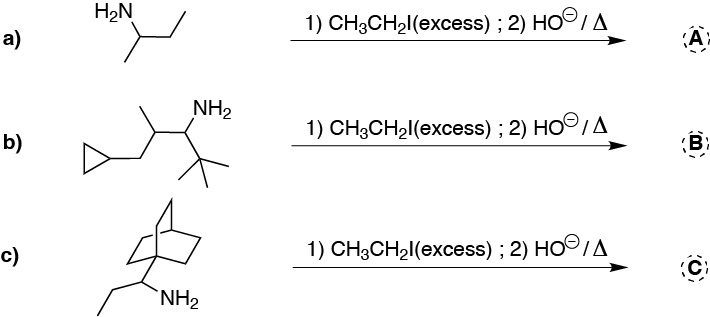### Problem 17)

Indicate which compounds (a-u) you would use in a Mannich reaction to obtain the compounds (I-III) in the box.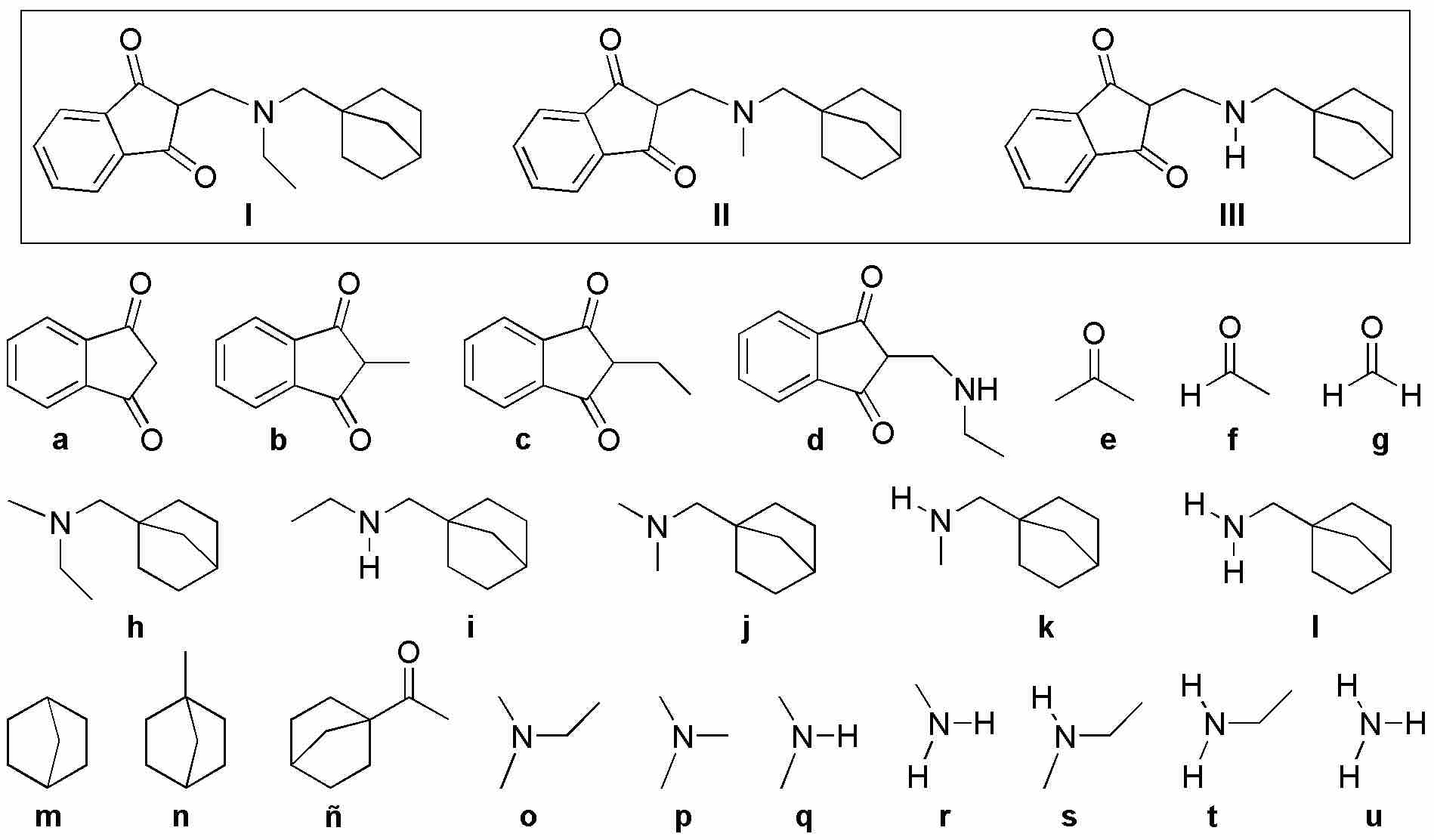### Problem 18)

Complete the following Gabriel syntheses (a-c).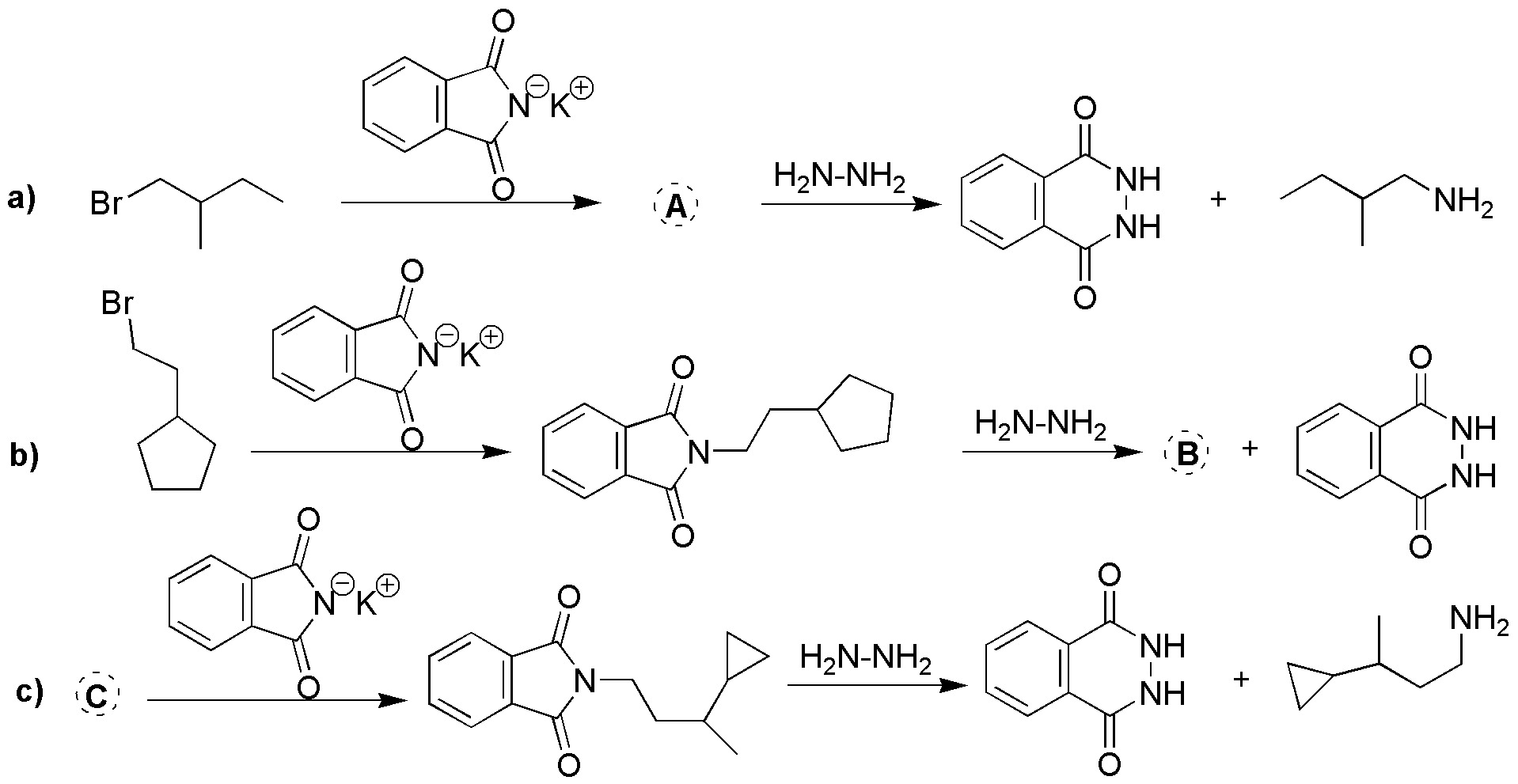### Problem 19)

What amines are obtained with the starting reagents (a-c), using Gabriel synthesis?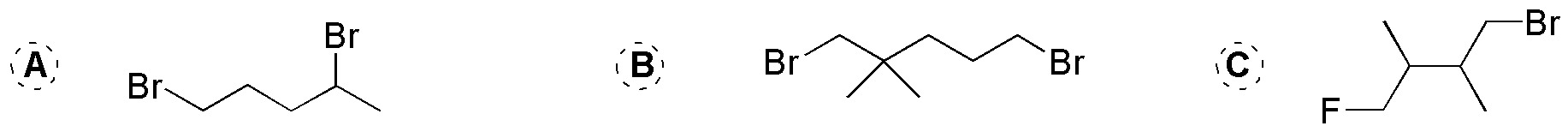### Problem 20)

Draw the structure of the compounds obtained when the following amines are treated with sodium nitrite in acidic medium.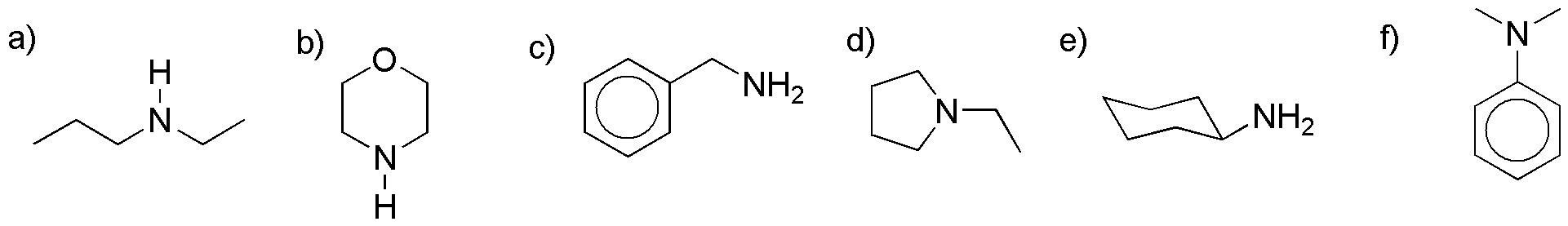### Problem 21)

How could the following transformations be carried out?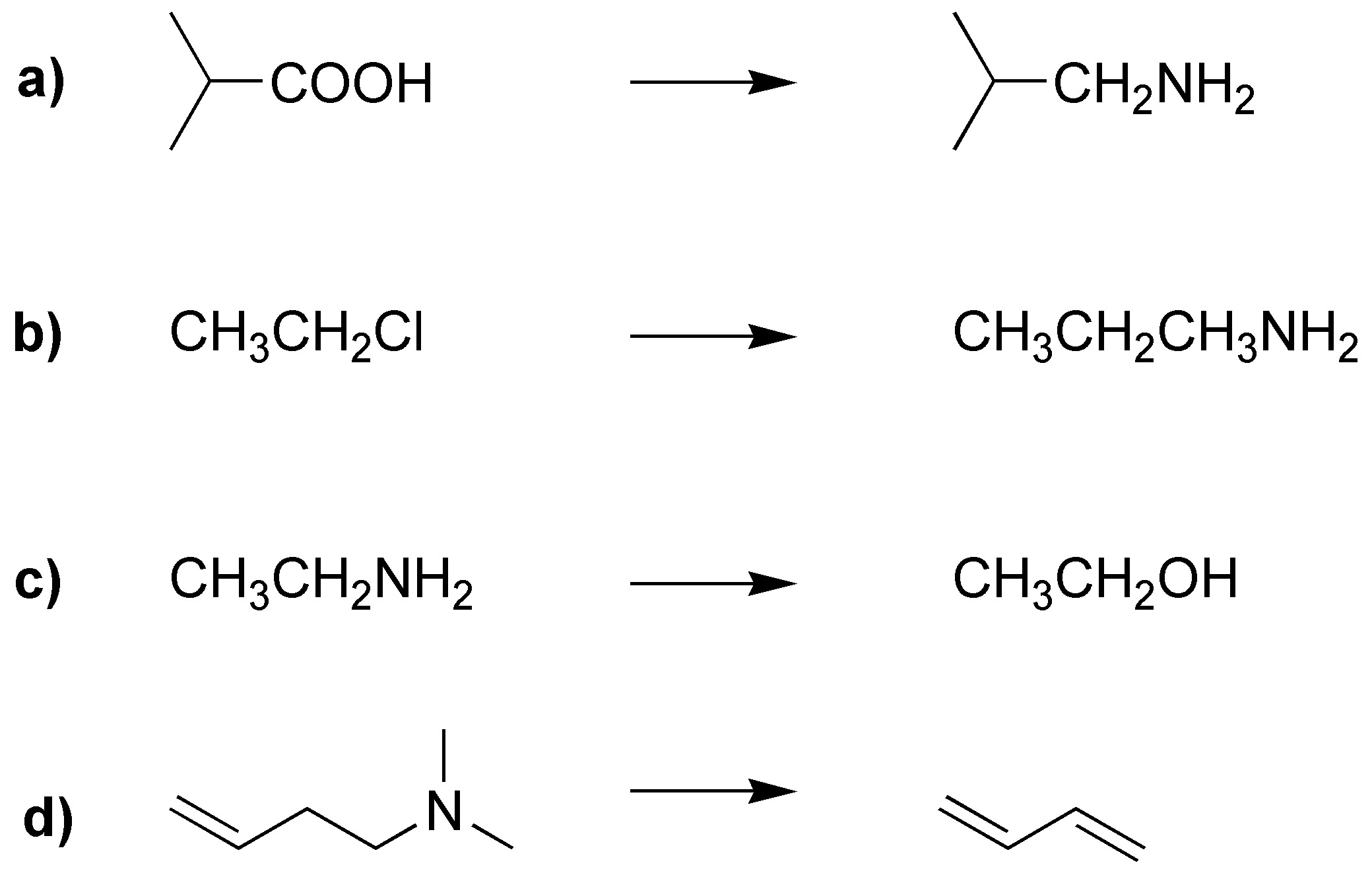### Problem 22)

What products would be obtained by Hofmann degradation of the following amines?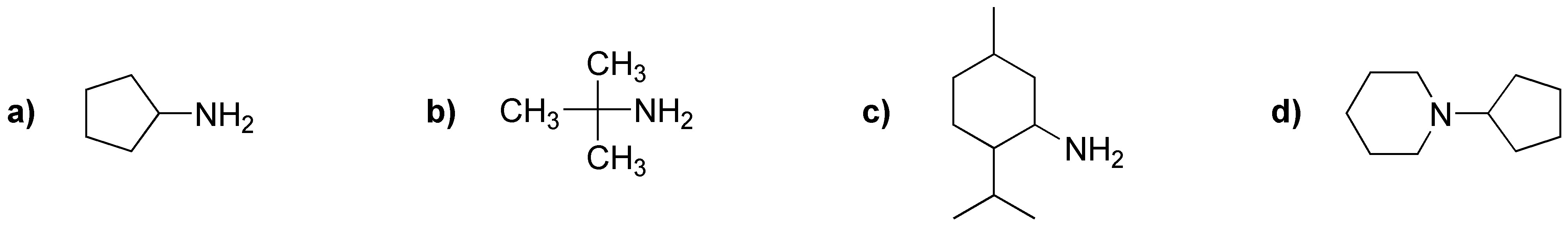### Problem 23)

Suggest a preparation of benzylamine using Gabriel synthesis.

Shares